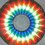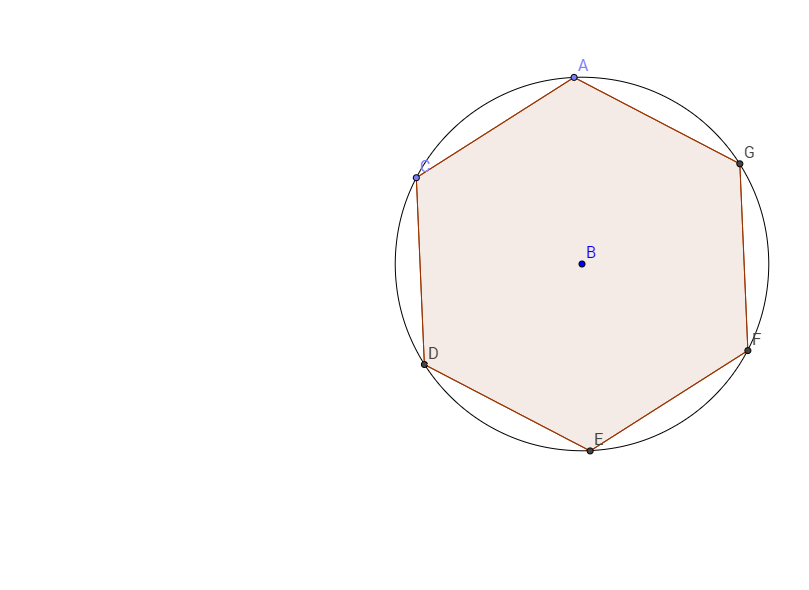# I'm back from my exams! Here are some questions.

If f(x)=$arcsin(\sin(\pi x))$.

Find

1. f(2.7)

2. f'(2.7)

3. $\int^{2.5}_0 {f(x)} {dx}$

If 1000 people are sitting around a circular table and each person makes a donation of the average of the two people sitting adjacent to him/her and it is known that one person made a contribution of 500 dollars, then find, if you can, the total amount donated and the maximum amount of money donated.

If $p(x)=(x+a_1)(x+a_2)...(x+a_{10})$ is a polynomial such that $\forall a_i \in R$ and all the 11 coefficients are positive, then answer true or false for the following questions:

1. p(x) has a global minimum.

2. $a_i$ are all positive.

3. p'(x) has all negative real roots.

4. p'(x) has all real roots.Note by Vishnu C
6 years ago

This discussion board is a place to discuss our Daily Challenges and the math and science related to those challenges. Explanations are more than just a solution — they should explain the steps and thinking strategies that you used to obtain the solution. Comments should further the discussion of math and science.

When posting on Brilliant:

• Use the emojis to react to an explanation, whether you're congratulating a job well done , or just really confused .
• Ask specific questions about the challenge or the steps in somebody's explanation. Well-posed questions can add a lot to the discussion, but posting "I don't understand!" doesn't help anyone.
• Try to contribute something new to the discussion, whether it is an extension, generalization or other idea related to the challenge.
• Stay on topic — we're all here to learn more about math and science, not to hear about your favorite get-rich-quick scheme or current world events.

MarkdownAppears as
*italics* or _italics_ italics
**bold** or __bold__ bold
- bulleted- list
• bulleted
• list
1. numbered2. list
1. numbered
2. list
Note: you must add a full line of space before and after lists for them to show up correctly
paragraph 1paragraph 2

paragraph 1

paragraph 2

[example link](https://brilliant.org)example link
> This is a quote
This is a quote
    # I indented these lines
# 4 spaces, and now they show
# up as a code block.

print "hello world"
# I indented these lines
# 4 spaces, and now they show
# up as a code block.

print "hello world"
MathAppears as
Remember to wrap math in $$ ... $$ or $ ... $ to ensure proper formatting.
2 \times 3 $2 \times 3$
2^{34} $2^{34}$
a_{i-1} $a_{i-1}$
\frac{2}{3} $\frac{2}{3}$
\sqrt{2} $\sqrt{2}$
\sum_{i=1}^3 $\sum_{i=1}^3$
\sin \theta $\sin \theta$
\boxed{123} $\boxed{123}$

## Comments

Sort by:

Top Newest

Okay, here's how you do the first one:

First draw the graph of the function. Although mathematically inaccurate, it's going to be a series of triangles with base on the x-axis.

2.7=2.5+0.2. When the arcsin function is applied, it is going to give you (0.5-0.2)pi=0.3pi

As the function has a slope = -PI at x=2.7.

The integral is pretty simple. It's the area under the curve. Height =PI/2 and base = 1/2 and there is one triangle like that because the positive and negative areas cancel out. And you get PI/8.

- 6 years ago

Log in to reply

@Brian Charlesworth , I could some help with the third one.

- 6 years ago

Log in to reply

The second one was actually modified a little bit, but the answer is still the same.Let A be the guy who donated 500 dollars. C must have donated 500+x and G must have donated 500-x, where x is any real number.With a little bit of simplification, you can see that D must have given 500+2x and F must have given 500-2x, and so on. The last guy, E, also gives 500 dollars. Because of symmetry, you can see that if you add it all up, you get 500*1000=500000.

Note that the maximum amount of money donated can never be found out because everyone could have given the same amount, i.e, x=0 is also a possible scenario.

- 6 years ago

Log in to reply

×

Problem Loading...

Note Loading...

Set Loading...